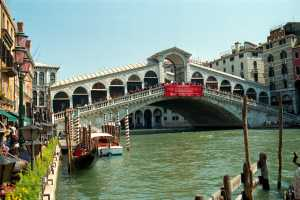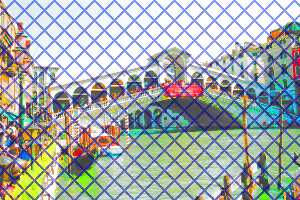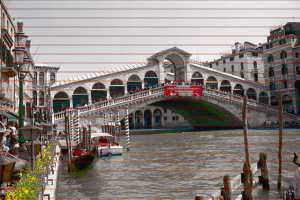Share your work!

On this page you invite you to send us tips and tricks, or images created with FilterFormula and share your experiences with other users. Please note that we do not take any warranties and we (ATS/Graphics) are not responsible for the correctness and any consequences of the filters shown on this page.

For the first contribution, we wish to thank Thomas Bernt for his great filter "Line Mill" - Enjoy!
(Please note that the filter is copyrighted by him). See the description within the source.

Some images created with this filter (plus original):

Original:2 Samples:The source is as follows (Use the "Cut & paste" feature to use it in FilterFormula).

 /*************************************************************** ** Line Mill - Part1  **************************************************************** * Copyright (C) 1999 by Thomas Bernt, all rights reserved.  **************************************************************** * These filter is provided free of charge and "as is" for your  * personal use.  * No warranties are made, express or implied. * This filter may not be distributed  without written  * authorization from the author.  **************************************************************** *  Rem: Slider 0[-255/255/3] means [range/range/default] * *  Slider 0 [0/255/2]    : Width of the lines in pixel *  Slider 1 [0/255/6]    : Width of the space between the lines *  Slider 2 [-255/255/0] : Adjust (shift) *  Slider 3 [0/255/0]    : Line transparency *  Slider 4 [-255/255/0] : Background light (L of the HLS system) *  Slider 5 [-255/255/0] : Background saturation *  Slider 6 [0/255/0]    : Effect 1 lines are only drawn when BG  *                          color is brighter then the slider value *  Slider 7 [0/255/0]    : Effect 2 the contrary to Effect 1 ************* *  Check box 1: Bit wise OR (|) between line and BG *  Check box 2: Bit wise AND (&) between line and BG *  Check box 3: Use the 2th user color as BG ************* *  Clr(1)    : Line color *  Clr(2)    : Color of the space between the lines *              (if Check box 3 is checked) ************* *  Selector  : 0/1/2/3/4/5 = *              horizontal / vertical / diagonal A *              diagonal B / mesh / diagonal mesh **************************************************************** ***************************************************************/ init { Arg1=Arg2=0; } // Arg2 only used for mesh drawing Pat=ctl(0)+ctl(1); // Pattern of one line + one space  if(sel(0)==0) Arg1=Arg2= y; //..........................horizontal if(sel(0)==1) Arg1=Arg2=  x; //.........................vertical if(sel(0)==2) Arg1=Arg2=  x+y; //.......................diagonal A     if(sel(0)==3) Arg1=Arg2= (x - y) + (X + Y); //..........diagonal B  if(sel(0)==4) { Arg1=x; Arg2=y;} //.................... mesh if(sel(0)==5) { Arg1=x+y; Arg2=(x - y) + (X + Y);} //...diagonal mesh if(!sel(0)) Arg1=Arg2= y; // make my day, execute this instruction ! //here we go, make the lines if( (Arg1+ctl(2)) % Pat < ctl(0) || (Arg2 + ctl(2)) % Pat < ctl(0) ) {   if(chk(0)) // FG AND BG    {     R=clr(0,0) | r;     G=clr(0,1) | g;     B=clr(0,2) | b;   }   else if(chk(1)) // FG OR BG   {     R=clr(0,0) & r;     G=clr(0,1) & g;     B=clr(0,2) & b;   }   else if (ctl(3)) // if slider 4 -> use the ff mix() function -> a*n/d + b(d-n)/d   {     R=( (r*ctl(3)/255) + ( clr(0,0)*(255-ctl(3))/ 255) );     G=( (g*ctl(3)/255) + ( clr(0,1)*(255-ctl(3))/ 255) );     B=( (b*ctl(3)/255) + ( clr(0,2)*(255-ctl(3))/ 255) );   }   else if (ctl(6))  // Effect 1   {    R=(( sub(r,g,b)+r)/2) >ctl(6)? clr(0,0) : r;    G=(( sub(r,g,b)+g)/2) >ctl(6)? clr(0,1) : g;    B=(( sub(r,g,b)+b)/2) >ctl(6)? clr(0,2) : b;   }   else if (ctl(7))  // Effect 2   {    R=(( sub(r,g,b) +r)/2)